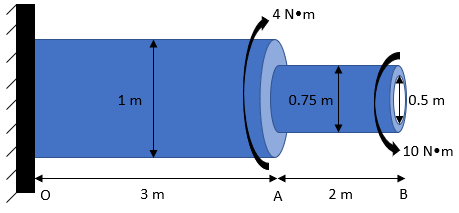## Torsion

In the below diagram, section OA is a solid titanium cylinder that is connected to section AB, which is a hollow steel pipe. What is the max shear stress in section AB?Hint
$$\tau =\frac{Tr}{J}$$$where $$\tau$$ is torsion, $$T$$ is the torque at the section of interest, $$r$$ is the radius to the point of interest, and $$J$$ is the section's polar moment of inertia Hint 2 Because we are interested in the polar moment of inertia of a hollow cylinder shaft: $$J_{hollow}=\frac{\pi (D_{outer}^{4}-D_{inner}^{4})}{32}$$$
where $$D$$ is the diameter.
Torsion stress in circular solid or thick-walled (t<0.1r) shafts:
$$\tau =\frac{Tr}{J}$$$where $$\tau$$ is torsion, $$T$$ is the torque at the section of interest, $$r$$ is the radius to the point of interest, and $$J$$ is the section's polar moment of inertia Because we are interested in the polar moment of inertia of a hollow cylinder shaft: $$J_{hollow}=\frac{\pi (D_{outer}^{4}-D_{inner}^{4})}{32}$$$
where $$D$$ is the diameter.
$$J=\frac{\pi ((0.75m)^{4}-(0.5m)^{4})}{32}=\frac{\pi(0.3164-0.0625)m^4}{32}=0.025\:m^4$$$Thus, $$\tau=\frac{10N\cdot m(\frac{0.75}{2}m)}{0.025\:m^4}=150\frac{N}{m^2}=150\:Pa$$$
150 Pa